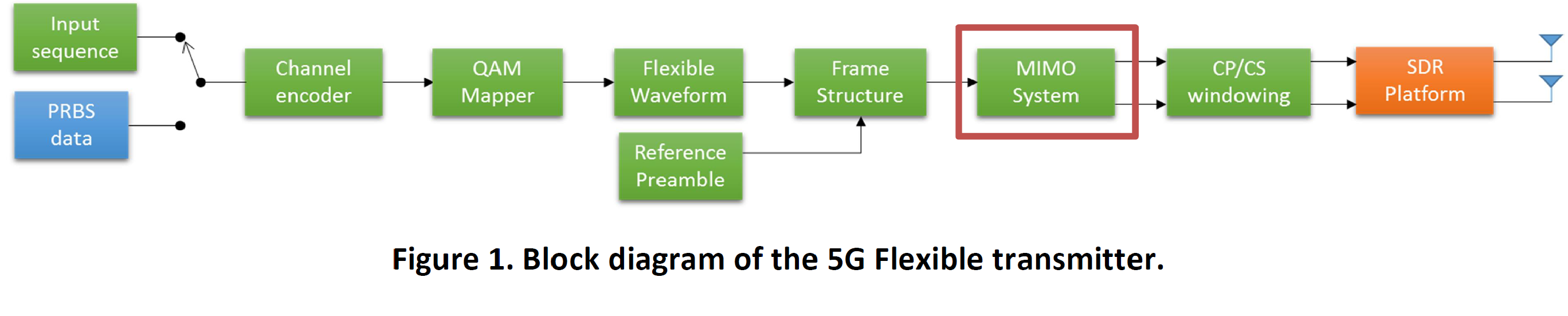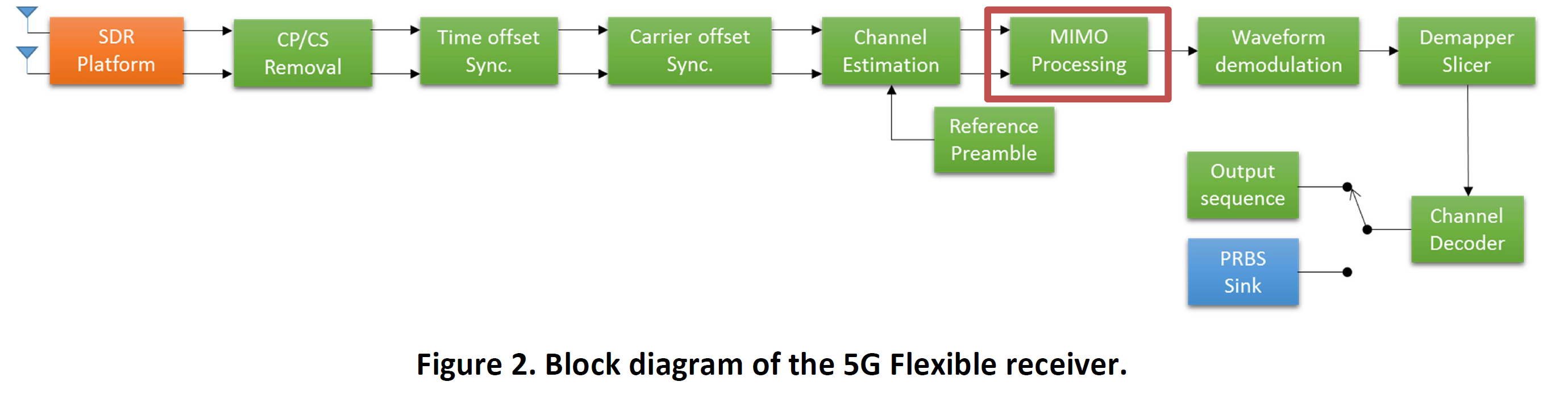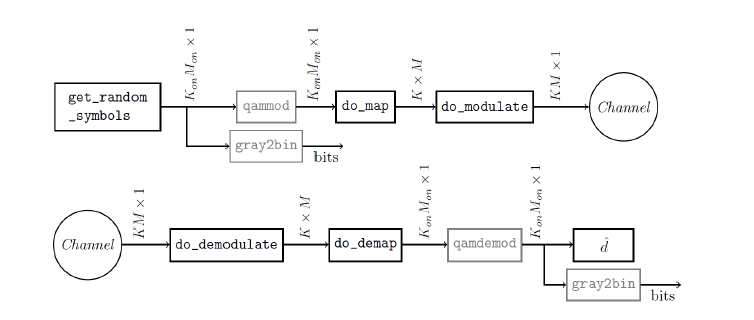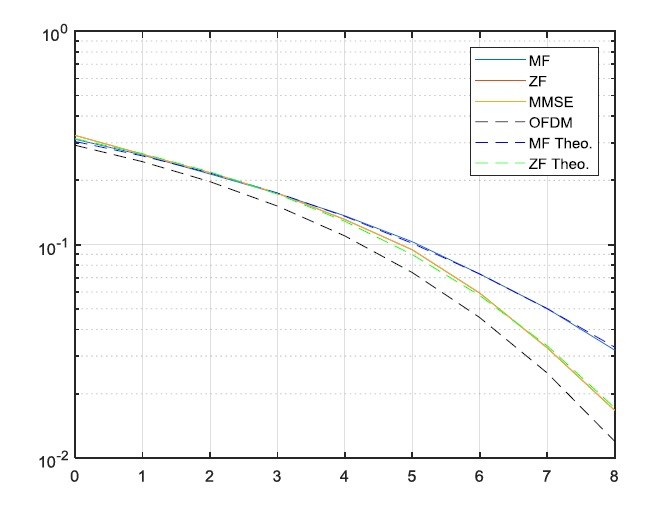# 5G Flexible Transceiver for Physical Layer Evaluation

## Basic overview

The aim of this proposal is to extend the flexible PHY software component made available by the eWINE project with MIMO technologies. The goal is to utilize the second independent RF chain of the USRP SDR to employ Alamouti space-time coding (STC) combined with GFDM. This technique, firstly introduced for OFDM based wireless transmission systems, increases the reliability of the data transfer by transmitting multiply copies of the data stream. However, it has not yet been applied on the GFDM flexible waveform generation framework and, since GFDM is a nonorthogonal waveform, its combination with STC is not a straight forward approach.## GFDM Library – How to use

In this library, only basic functions for GFDM transmission, signal generation, and naive reception are included, nothing fancy like channel estimation/equalization, iterative receivers, etc. The functions are grouped into several parts:

• get_* These functions return objects that are commonly used for GFDM simulations.
• calc_* These functions calculate (not only) performance values based on the passed objects.
• do_* These more complex functions do actual simulation steps.

Remark: To use the library functions, you must include the library folder and subfolders to the MATLAB path.

The GFDM library has a folder with examples of use for the functions (GFDM_libraryexamples). With the purpose of illustration, an example file (GFDM_libraryexamplesser_in_awgn.m) for generation of a GFDM SER (Symbol Error Rate) curve is detailed below.

For the GFDM configuration, a parameter structure p contains the configuration of the GFDM system.

```% GFDM configuration
p = get_defaultGFDM('BER');
p.pulse = 'rc_fd';
p.a = 0.5;```### GFDM Transmitter

1. Create random data symbols
```% create symbols
s = get_random_symbols(p);```

The function get_random_symbols(p) returns a sequence of integers in range 0 ⋯ 2????−1 which are the transmitted data symbols.

1. QAM-Modulate the symbols to QAM symbols and map the symbol stream to the data matrix
```% map them to qam and to the D matrix
D = do_map(p, do_qammodulate(s, p.mu));```

Map the integers in range 0 ⋯ 2????−1 to a quadratic QAM modulation using the MATLAB function qammod. The one-dimensional symbol stream is mapped to the data matrix D according to the values of ???????????????? and ????????????????. Empty sub-carriers and sub-symbols in D are set to zero. Afterwards there is the possibility to insert specific pilots or other information in the slots where no data is present.

1. GFDM-Modulate the data matrix
`x = do_modulate(p, D);`

The matrix ???? is processed with the GFDM modulation scheme to produce a time domain signal with length ???????? that can be processed further. The transmitted signal is applied to an AWGN channel using the function do_channel. A channel object from MATLAB can be used as input parameter for this function for different channels.

```% channel -> AWGN
xch = do_channel(x, 1, snr(si));% channel -> AWGN
xch = do_channel(x, 1, snr(si));```